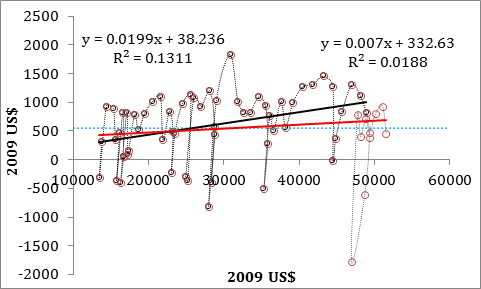## On Decreasing Rate Of Economic Growth In The USA

We published a few papers [e.g., 123] on economic growth. There are numerous plots demonstrating the decelerating rate of real economic growth in developed countries. The long-term component of real GDP increase is very simple – annual increment in real GDP per capita, rGDPpc, is a constant, which does not change with time since the late 1940s. This makes the rate of growth to be inversely proportional to the current level of rGDPpc: dln(rGDPpc(t)) = A/rGDPpc(t). The population component is large in the USA – approximately 1% per year since the 1950s, but negative in Japan. Therefore, we use rGDPpc instead of rGDP, which is rather misleading.

In the first paper published in 2005, we used data between 1950 and 2003 and found that the rGDPpc annual increment has a positive slope. It was considered as an indication of future problems between 2004 and 2016, which was actually observed as the Great Recession and further sluggish recovery. Figure 1 displays the original time series between 1947 and 2003 (black circles) extended by the estimated made since 2004. Instead of time, we use rGDPpc as argument. For recession periods, when the rGDPpc falls, one observes loops in the curve. Our prediction was right – the slope of the regression line has dropped from 0.02 (black linear trend line) to 0.007 (red trend line). The average increment in rGDPpc was \$551 as measured  in 2009US\$.Figure 1. Annual increment in real GDP per capita in the USA since 1947. Black circles show the period between 1947 and 2003, and red circles show the period extended into 2016. Linear trends are shown in black and red, respectively. Two regression equations demonstrate the change in slope since 2003.

There is another problem with the original rGDPpc time series borrowed from the BEA web site. It is calculated as a ratio of rGDP and total population, while only economically active population matters. Therefore, we have to correct the originalrGDPpc for the ratio shown in Figure 2. When the rGDPpc divided by the population correction factor, one obtains a better view on the long-term growth rate, as depicted in Figure 3. The trend in the rGDPpc annual increment is absent. This observation means that the rate of real GDP growth in the USA (and other developed countries) is decaying inversely proportional to rGDPpc. Leading economic countries will be growing at a rate of about 1.5% per year in the next 20 years. In 2016, the rate of rGDPpc growth was 0.9% per year. This mediocre growth will be accompanied by decreasing rate of population growth as observed since the earlier 2000s.

Disclosure: None.

How did you like this article? Let us know so we can better customize your reading experience. Users' ratings are only visible to themselves.

#### CommentsLeave a comment to automatically be entered into our contest to win a free Echo Show.